#### General configuration spaces

Velocity constraints can be considered in the same way on a general C-space. Assume thatis a smooth manifold (a manifold was not required to be smooth in Chapter 4 because derivatives were not needed there). All constraints are expressed using a coordinate neighborhood, as defined in Section 8.3.2. For expressing differential models, this actually makes an-dimensional manifold look very much like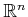. It is implicitly understood that a change of coordinates may occasionally be needed; however, this does not complicate the expression of constraints. This makes it possible to ignore many of the manifold technicalities and think about the constraints as if they are applied to.

Now consider placing velocity constraints on. Imagine how complicated velocity constraints could become if any semi-algebraic model is allowed. Velocity constraints oncould be as complicated as any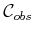. It is not even necessary to use algebraic primitives. In general, the constraints can be expressed as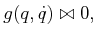(13.1)

in which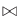could once again be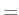,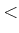,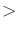,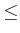, or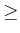. The same expressive power can be maintained even after eliminating some of these relations. For example, any constraint of the form (13.1) can be expressed as a combination of constraints of the form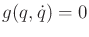and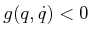. All of the relations are allowed here, however, to make the formulations simpler.

Constraints expressed in the form shown in (13.1) are called implicit. As explained in Chapters 3 and 4, it can be very complicated to obtain a parametric representation of the solutions of implicit equations. This was seen, for example, in Section 4.4, in which it was difficult to characterize the set of configurations that satisfy closure constraints. Nevertheless, we will be in a much better position in terms of developing planning algorithms if a parametric representation of the constraints can be obtained. Fortunately, most constraints that are derived from robots, vehicles, and other mechanical systems can be expressed in parametric form.

Steven M LaValle 2020-08-14